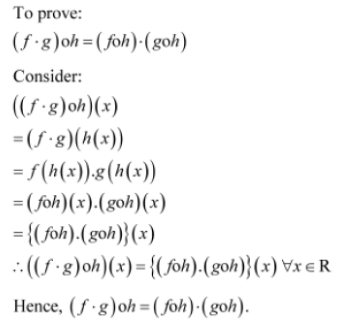# Let f, g and h be functions from R to R. Show that: (f + g) oh = foh + goh

Let f, g and h be functions from R to R. Show that:
(f + g) oh = foh + goh
(f.g) oh = (foh).(goh)